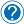# PHP math operations and operators

## PHP math operations and operators

PHP is a very powerful language that can help you to perform mathematical operations really easy. Math expressions are made up of operators and operands. You can perform easy operations like adding, subtracting, multiplying or dividing with PHP. Even comparison operators can be used with this language (greater than, less than, equal with).

Let’s see some examples with possible operation that you can perform in PHP.

## Comparison operations

Note: In php, as in many languages, one equal ( = ) means to assign something, two equals ( == ) means to compare their values, and three equals ( === ) means to compare their values and also their type.

## Other types of operations

Rounding numbers:

In PHP you can round numbers in three different ways. One is the classic one, in which everything that is .5 or over will be rounded up. You can also use ceil to round  everything up, or floor to round everything down.

Sine, cosine or tangent

The Sin () function is a mathematical function used to calculate the sine of a given number.
The Cos () Function, calculates the cosine of the given number and returns it in radians.
The Tan () function is a mathematical function used to calculate the tangent of a given number. The result is returned in radians.

Number formatting

Min or max

Min – If the first and only parameter is an array, min() returns the lowest value in that array. If at least two parameters are provided, min() returns the smallest of these values.

Max – If the first and only parameter is an array, max() returns the highest value in that array. If at least two parameters are provided, max() returns the biggest of these values.

## Summary

PHP is a very easy to use language when it comes to math. It is very suggestive and yet powerful. There are plenty of other things that you can perform in different situations.  We covered only a few of them here in this article, for the sake of enumerating few possibilities that you have with this language.Request an article ←